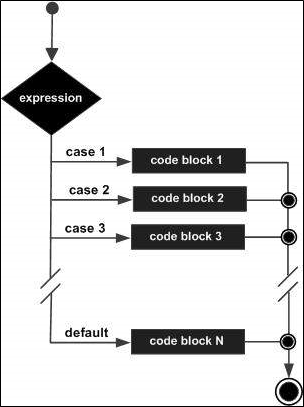# Difference Between Algorithm and Flowchart

Analysis of AlgorithmsAlgorithmsMisc Algorithms

In this post, let us understand the differences between a flowchart and an algorithm.

## Algorithm

• It is defined as a sequence of well-defined steps.
• These steps provide a solution/ a way to solve a problem in hand.
• It is a systematic, and a logical approach, where the procedure is defined step-wise.
• It gives the solution to a specific problem.
• This solution would be translated to machine code, which is then executed by the system to give the relevant output.
• Many simple operations are combined to help form a more complicated operation, which is performed with ease by the computer.
• Algorithms can be represented using natural language, flowchart and so on.
• It is difficult to understand.
• Plain text is used.
• It is easy to debug.
• Its construction is tough.
• There are no rules to follow while constructing it.
• It can be understood as the pseudocode for a program.

Algorithm for linear search

• Start searching for an element from the left-most part of the array.
• Compare one element every iteration with the item_to_be_searched.
• If no matches are found, return -1.
• Otherwise, return the index at which the element is present.

## Flowchart

• It is a graphical representation of an algorithm.
• Programmers use it as a program-planning tool in order to solve a problem.
• It uses symbols that are inter-connected.
• This will help indicate the flow of control and information, and processing.
• The process of drawing a flowchart for an algorithm is known as "flowcharting".
• It is a diagram that can be created using different shapes to represent flow of data.
• It is easy to understand.
• It is difficult to debug.
• It is easy to build.
• Certain riles are to be followed while building it.
• It is a graphical representation of logic.

Flowchart for Switch Statement